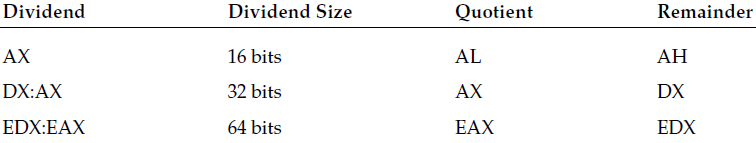﻿ notes12
/* personal notes of renzo diomedi */

~ 00001100 ~

if you divide 9 by 2 , this produces two parts to the answer. The quotient is the number of times the divisor goes into the dividend. The remainder is how much is left over (the fractional part of the answer). The division instructions produce both the quotient and remainder parts as results of the division.

DIV (unsigned) and IDIV (signed) instructions are used for integer division.

Unsigned division

The DIV instruction is used for dividing unsigned integers. The format of the DIV instruction is div divisor

where divisor is the value that is divided into the implied dividend, and can be an 8-, 16-, or 32-bit register or value in memory. The dividend must already be stored in the AX register (for a 16-bit value), the DX:AX register pair (for a 32-bit value), or the EDX:EAX register pair (for a 64-bit value) before the DIV instruction is performed.
The maximum value allowed for the divisor depends on the size of the dividend. For a 16-bit dividend, the divisor can only be 8 bits, for a 32-bit dividend 16 bits, and for a 64-bit dividend the divisor can only be 32 bits.
The result of the division is two separate numbers: the quotient and the remainder. Both values are stored in the same registers used for the dividend value.This means that you will LOSE the value of the dividend when the division completes.

Then the result will alter the value of the DX or EDX registers

divtest2.s

divtest2.o

divtest2.exe # windows environment

C:\>gdb -q users\rnz\desktop\divtest2.exe
(gdb) break 2
Breakpoint 1 at 0x401000: file users\rnz\desktop\divtest2.s, line 2.
(gdb) run
Starting program: C:\users\rnz\desktop\divtest2.exe
Breakpoint 1, ?? () at users\rnz\desktop\divtest2.s:14
14 nop
(gdb) s
15 movb dividend, %al
(gdb) s
16 movb dividend+1, %ah
(gdb) s
17 divb divisor
(gdb) s
18 movb %al, quotient
(gdb) s
19 movb %ah, remainder
(gdb) print \$ah
\$1 = 1
(gdb) print \$al
\$2 = 4
(gdb) print \$ax
\$3 = 260 # 256+4

Signed division

The IDIV instruction is used exactly like the DIV instruction, but for dividing signed integers. It too uses an implied dividend, located in the AX register, the DX:AX register pair, or the EDX:EAX register pair.
Unlike the IMUL instruction, there is only one format for the IDIV instruction, which specifies the divisor used in the division:

idiv divisor

where divisor can again be an 8-, 16-, or 32-bit register or value in memory.

For signed integer division, the sign of the remainder is always the sign of the dividend.

the size of the dividend must be twice the size of the divisor

The biggest problem with integer division is detecting when an error condition has occurred, such as when a division by zero happens, or the quotient (or remainder) overflows the destination register. When an error occurs, the system produces an interrupt, which will produce an error on the Linux system,

\$ ./progname
Floating point exception